Download Presentation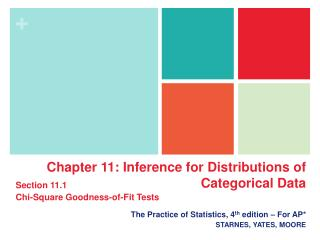The Practice of Statistics, 4 th edition – For AP* STARNES, YATES, MOORE

# The Practice of Statistics, 4 th edition – For AP* STARNES, YATES, MOORE - PowerPoint PPT Presentation

Chapter 11: Inference for Distributions of Categorical Data. Section 11.1 Chi-Square Goodness-of-Fit Tests. The Practice of Statistics, 4 th edition – For AP* STARNES, YATES, MOORE. Chapter 11 Inference for Distributions of Categorical Data. 11.1 Chi-Square Goodness-of-Fit TestsI am the owner, or an agent authorized to act on behalf of the owner, of the copyrighted work described.
Download Presentation## The Practice of Statistics, 4 th edition – For AP* STARNES, YATES, MOORE

An Image/Link below is provided (as is) to download presentation

Download Policy: Content on the Website is provided to you AS IS for your information and personal use and may not be sold / licensed / shared on other websites without getting consent from its author.While downloading, if for some reason you are not able to download a presentation, the publisher may have deleted the file from their server.

- - - - - - - - - - - - - - - - - - - - - - - - - - E N D - - - - - - - - - - - - - - - - - - - - - - - - - -
Presentation Transcript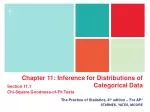Chapter 11: Inference for Distributions of Categorical Data

Section 11.1

Chi-Square Goodness-of-Fit Tests

The Practice of Statistics, 4th edition – For AP*

STARNES, YATES, MOORE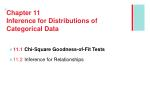Chapter 11Inference for Distributions of Categorical Data
• 11.1 Chi-Square Goodness-of-Fit Tests
• 11.2 Inference for Relationships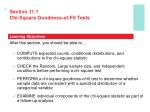Section 11.1Chi-Square Goodness-of-Fit Tests

Learning Objectives

After this section, you should be able to…

• COMPUTE expected counts, conditional distributions, and contributions to the chi-square statistic
• CHECK the Random, Large sample size, and Independent conditions before performing a chi-square test
• PERFORM a chi-square goodness-of-fit test to determine whether sample data are consistent with a specified distribution of a categorical variable
• EXAMINE individual components of the chi-square statistic as part of a follow-up analysis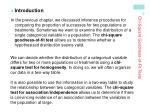Chi-Square Goodness-of-Fit Tests
• Introduction

In the previous chapter, we discussed inference procedures for comparing the proportion of successes for two populations or treatments. Sometimes we want to examine the distribution of a single categorical variable in a population. The chi-square goodness-of-fit test allows us to determine whether a hypothesized distribution seems valid.

We can decide whether the distribution of a categorical variable differs for two or more populations or treatments using a chi-square test for homogeneity. In doing so, we will often organize our data in a two-way table.

It is also possible to use the information in a two-way table to study the relationship between two categorical variables. The chi-square test for association/independence allows us to determine if there is convincing evidence of an association between the variables in the population at large.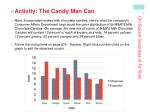Chi-Square Goodness-of-Fit Tests
• Activity: The Candy Man Can
• Mars, Incorporated makes milk chocolate candies. Here’s what the company’s Consumer Affairs Department says about the color distribution of its M&M’S Milk Chocolate Candies: On average, the new mix of colors of M&M’S Milk Chocolate Candies will contain 13 percent of each of browns and reds, 14 percent yellows, 16 percent greens, 20 percent oranges and 24 percent blues.
• Follow the instructions on page 676. Teacher: Right-click (control-click) on the graph to edit the observed counts.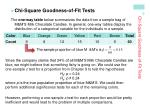Chi-Square Goodness-of-Fit Tests
• Chi-Square Goodness-of-Fit Tests

The one-way table below summarizes the data from a sample bag of M&M’S Milk Chocolate Candies. In general, one-way tables display the distribution of a categorical variable for the individuals in a sample.

Since the company claims that 24% of all M&M’S Milk Chocolate Candies are blue, we might believe that something fishy is going on. We could use the one-sample z test for a proportion from Chapter 9 to test the hypotheses

H0: p = 0.24

Ha: p ≠ 0.24

where p is the true population proportion of blue M&M’S. We could then perform additional significance tests for each of the remaining colors.

However, performing a one-sample z test for each proportion would be pretty inefficient and would lead to the problem of multiple comparisons.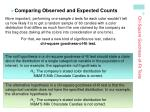More important, performing one-sample z tests for each color wouldn’t tell us how likely it is to get a random sample of 60 candies with a color distribution that differs as much from the one claimed by the company as this bag does (taking all the colors into consideration at one time).

For that, we need a new kind of significance test, called a

chi-square goodness-of-fit test.

Chi-Square Goodness-of-Fit Tests
• Comparing Observed and Expected Counts

The null hypothesis in a chi-square goodness-of-fit test should state a claim about the distribution of a single categorical variable in the population of interest. In our example, the appropriate null hypothesis is

H0: The company’s stated color distribution for

M&M’S Milk Chocolate Candies is correct.

The alternative hypothesis in a chi-square goodness-of-fit test is that the categorical variable does not have the specified distribution. In our example, the alternative hypothesis is

Ha: The company’s stated color distribution for

M&M’S Milk Chocolate Candies is not correct.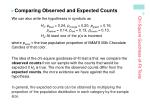We can also write the hypotheses in symbols as

H0: pblue= 0.24, porange= 0.20, pgreen= 0.16,

pyellow= 0.14, pred= 0.13, pbrown= 0.13,

Ha: At least one of the pi’s is incorrect

where pcolor = the true population proportion of M&M’S Milk Chocolate Candies of that color.

Chi-Square Goodness-of-Fit Tests
• Comparing Observed and Expected Counts

The idea of the chi-square goodness-of-fit test is this: we compare the observed counts from our sample with the counts that would be expected if H0is true. The more the observed counts differ from the expected counts, the more evidence we have against the null hypothesis.

In general, the expected counts can be obtained by multiplying the proportion of the population distribution in each category by the sample size.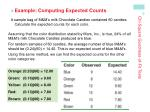Chi-Square Goodness-of-Fit Tests
• Example: Computing Expected Counts

A sample bag of M&M’s milk Chocolate Candies contained 60 candies. Calculate the expected counts for each color.

Assuming that the color distribution stated by Mars, Inc., is true, 24% of all M&M’s milk Chocolate Candies produced are blue.

For random samples of 60 candies, the average number of blue M&M’s should be (0.24)(60) = 14.40. This is our expected count of blue M&M’s.

Using this same method, we can find the expected counts for the other color categories:

Orange: (0.20)(60) = 12.00

Green: (0.16)(60) = 9.60

Yellow: (0.14)(60) = 8.40

Red: (0.13)(60) = 7.80

Brown: (0.13)(60) = 7.80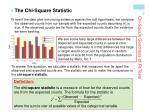Chi-Square Goodness-of-Fit Tests
• The Chi-Square Statistic

To see if the data give convincing evidence against the null hypothesis, we compare the observed counts from our sample with the expected counts assuming H0is true. If the observed counts are far from the expected counts, that’s the evidence we were seeking.

We see some fairly large differences between the observed and expected counts in several color categories. How likely is it that differences this large or larger would occur just by chance in random samples of size 60 from the population distribution claimed by Mars, Inc.?

To answer this question, we calculate a statistic that measures how far apart the observed and expected counts are. The statistic we use to make the comparison is the chi-square statistic.

Definition:

The chi-square statistic is a measure of how far the observed counts are from the expected counts. The formula for the statistic isChi-Square Goodness-of-Fit Tests
• Example: Return of the M&M’s

The table shows the observed and expected counts for our sample of 60 M&M’s Milk Chocolate Candies. Calculate the chi-square statistic.Chi-Square Goodness-of-Fit Tests
• The Chi-Square Distributions and P-Values

The Chi-Square Distributions

The chi-square distributions are a family of distributions that take only positive values and are skewed to the right. A particular chi-square distribution is specified by giving its degrees of freedom. The chi-square goodness-of-fit test uses the chi-square distribution with degrees of freedom = the number of categories - 1.Chi-Square Goodness-of-Fit Tests
• Example: Return of the M&M’s

Since our P-value is between 0.05 and 0.10, it is greater than α = 0.05.Therefore, we fail to reject H0. We don’t have sufficient evidence to conclude that the company’s claimed color distribution is incorrect.Chi-Square Goodness-of-Fit Tests
• Carrying Out a Test

Before we start using the chi-square goodness-of-fit test, we have two important cautions to offer.

1. The chi-square test statistic compares observed and expected counts. Don’t try to perform calculations with the observed and expected proportions in each category.

2. When checking the Large Sample Size condition, be sure to examine the expected counts, not the observed counts.

• The chi-square goodness-of-fit test uses some approximations that become more accurate as we take more observations. Our rule of thumb is that all expected counts must be at least 5. This Large Sample Size condition takes the place of the Normal condition for z and t procedures. To use the chi-square goodness-of-fit test, we must also check that the Random and Independent conditions are met.
• Conditions: Use the chi-square goodness-of-fit test when
• Random The data come from a random sample or a randomized experiment.
• Large Sample Size All expected counts are at least 5.
• Independent Individual observations are independent. When sampling without replacement, check that the population is at least 10 times as large as the sample (the 10% condition).

The Chi-Square Goodness-of-Fit Test

Suppose the Random, Large Sample Size, and Independent conditions are

met. To determine whether a categorical variable has a specified distribution, expressed as the proportion of individuals falling into each possible category, perform a test of

H0:The specified distribution of the categorical variable is correct.

Ha: The specified distribution of the categorical variable is not correct.

We can also write these hypotheses symbolically using pito represent the

proportion of individuals that fall in category i:

H0: p1 = ___, p2 = ___, …, pk = ___.

Ha: At least one of the pi’s is incorrect.

Start by finding the expected count for each category assuming that H0is

true. Then calculate the chi-square statisticChi-Square Goodness-of-Fit Tests
• Example: When Were You Born?

Are births evenly distributed across the days of the week? The one-way table below shows the distribution of births across the days of the week in a random sample of 140 births from local records in a large city. Do these data give significant evidence that local births are not equally likely on all days of the week?

State: We want to perform a test of

H0: Birth days in this local area are evenly distributed across the days of the week.

Ha: Birth days in this local area are not evenly distributed across the days of the week.

The null hypothesis says that the proportions of births are the same on all days. In that case, all 7 proportions must be 1/7. So we could also write the hypotheses as

H0: pSun = pMon = pTues = . . . = pSat = 1/7.

Ha: At least one of the proportions is not 1/7.

We will use α = 0.05.

Plan: If the conditions are met, we should conduct a chi-square goodness-of-fit test.

• Random The data came from a random sample of local births.

• Large Sample Size Assuming H0is true, we would expect one-seventh of the births to occur on each day of the week. For the sample of 140 births, the expected count for all 7 days would be 1/7(140) = 20 births. Since 20 ≥ 5, this condition is met.

• Independent Individual births in the random sample should occur independently (assuming no twins). Because we are sampling without replacement, there need to

be at least 10(140) = 1400 births in the local area. This should be the case in a large city.Chi-Square Goodness-of-Fit Tests
• Example: When Were You Born?

Do: Since the conditions are satisfied, we can perform a chi-square goodness-of-fit test. We begin by calculating the test statistic.

P-Value:

Using Table C: χ2 = 7.60 is less than the smallest entry in the df = 6 row, which corresponds to tail area 0.25. The P-value is therefore greater than 0.25.

Using technology: We can find the exact P-value with a calculator: χ2cdf(7.60,1000,6)= 0.269.

Conclude: Because the P-value, 0.269, is greater than α = 0.05, we fail to reject H0. These 140 births don’t provide enough evidence to say that all local births in this area are not evenly distributed across the days of the week.Chi-Square Goodness-of-Fit Tests
• Example: Inherited Traits

Biologists wish to cross pairs of tobacco plants having genetic makeup Gg, indicating that each plant has one dominant gene (G) and one recessive gene (g) for color. Each offspring plant will receive one gene for color from each parent.

The Punnett square suggests that the expected ratio of green (GG) to yellow-green (Gg) to albino (gg) tobacco plants should be 1:2:1.

In other words, the biologists predict that 25% of the offspring will be green, 50% will be yellow-green, and 25% will be albino.

To test their hypothesis about the distribution of offspring, the biologists mate 84 randomly selected pairs of yellow-green parent plants.

Of 84 offspring, 23 plants were green, 50 were yellow-green, and 11 were albino.

Do these data differ significantly from what the biologists have predicted? Carry out an appropriate test at the α = 0.05 level to help answer this question.Chi-Square Goodness-of-Fit Tests
• Example: Inherited Traits

State: We want to perform a test of

H0: The biologists’ predicted color distribution for tobacco plant offspring is correct.

That is, pgreen= 0.25, pyellow-green = 0.5, palbino= 0.25

Ha: The biologists’ predicted color distribution isn’t correct. That is, at least one of the stated proportions is incorrect.

We will use α = 0.05.

Plan: If the conditions are met, we should conduct a chi-square goodness-of-fit test.

• Random The data came from a random sample of local births.

• Large Sample Size We check that all expected counts are at least 5. Assuming H0is true, the expected counts for the different colors of offspring are

green: (0.25)(84) = 21; yellow-green: (0.50)(84) = 42; albino: (0.25)(84) = 21

The complete table of observed and expected counts is shown below.

• Independent Individual offspring inherit their

traits independently from one another. Since

we are sampling without replacement, there

would need to be at least 10(84) = 840

tobacco plants in the population. This seems

reasonable to believe.Chi-Square Goodness-of-Fit Tests
• Example: Inherited Traits

Do: Since the conditions are satisfied, we can perform a chi-square goodness-of-fit test. We begin by calculating the test statistic.

P-Value:

Note that df = number of categories - 1 = 3 - 1 = 2. Using df = 2, the P-value from the calculator is 0.0392

Conclude: Because the P-value, 0.0392, is less than α = 0.05, we will reject H0. We have convincing evidence that the biologists’ hypothesized distribution for the color of tobacco plant offspring is incorrect.In the chi-square goodness-of-fit test, we test the null hypothesis that a categorical variable has a specified distribution. If the sample data lead to a statistically significant result, we can conclude that our variable has a distribution different from the specified one.

When this happens, start by examining which categories of the variable show large deviations between the observed and expected counts.

Then look at the individual terms that are added together to produce the test statistic χ2. These components show which terms contribute most to the chi-square statistic.

Chi-Square Goodness-of-Fit Tests
• Follow-up AnalysisSection 11.1Chi-Square Goodness-of-Fit Tests

Summary

In this section, we learned that…

• A one-way table is often used to display the distribution of a categorical variable for a sample of individuals.
• The chi-square goodness-of-fit test tests the null hypothesis that a categorical variable has a specified distribution.
• This test compares the observed count in each category with the counts that would be expected if H0were true. The expected count for any category is found by multiplying the specified proportion of the population distribution in that category by the sample size.
• The chi-square statistic isSection 11.1Chi-Square Goodness-of-Fit Tests

Summary

• The test compares the value of the statistic χ2with critical values from the chi-square distribution with degrees of freedom df = number of categories - 1. Large values of χ2are evidence against H0, so the P-value is the area under the chi-square density curve to the right of χ2.
• The chi-square distribution is an approximation to the sampling distribution of the statistic χ2. You can safely use this approximation when all expected cell counts are at least 5 (Large Sample Size condition).
• Be sure to check that the Random, Large Sample Size, and Independent conditions are met before performing a chi-square goodness-of-fit test.
• If the test finds a statistically significant result, do a follow-up analysis that compares the observed and expected counts and that looks for the largest components of the chi-square statistic.